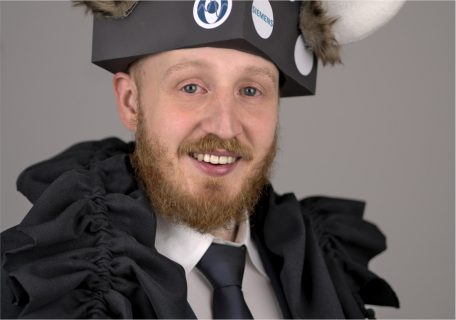# Tobias WürflPhotography by Alexander Preuhs

## Applications of Data Consistency Conditions in Cone-Beam Computed Tomography

The mathematical model of computed tomography data acquisition shows that every such measurement contains a degree of redundancy. This redundancy can be used to formulate data consistency conditions which enable quantifying how accurately a measurement fulﬁlls the mathematical model. This enables compensating for inaccuracies in the measurement process by formulating artifact compensation as optimization problems of an objective function based on such data consistency conditions.

In the course of this thesis, various methods to deal with diﬀerent types of such inaccuracies in the measurement have been proposed. One type of inaccuracy arises due to the simpliﬁed physical model of computed tomography reconstruction which assumes a mono-chromatic X-ray source. This leads to an eﬀect commonly named beam hardening. To compensate artifacts from this beam hardening eﬀect a projection-based algorithm named the ECC2 algorithm is introduced. It uses a recently proposed data consistency condition called the Grangeat data consistency condition to formulate an eﬃcient procedure to estimate parameters of an appropriate compensation model. This compensation model is derived to be physically plausible by mathematical analysis of the beam hardening eﬀect.

This method is extended in a second algorithm named the MECC2 algorithm to incorporate the possibility to compensate artifacts caused by the diﬀerent energy dependencies of the linear attenuation coeﬃcient of diﬀerent materials. Eﬃcient parameter estimation techniques and methods for physically plausible constraints on the compensation function are also presented.

Another category of inaccuracies in the measurement are caused by inaccurate knowledge of the acquisition geometry or rigid movement of the imaged object. A general strategy to solve both problems is to estimate the geometry of the acquisition projection-based. However, this is diﬃcult for X-ray imaging modalities because standard techniques from computer vision can not be translated straightforward to this setting. This is caused by the fact, that computer vision algorithms rely on identifying corresponding points in multiple pictures of the same scene as a fundamental step. This is very hard in X-ray imaging due to the diﬀerent image formation model. To solve this issue a method to estimate the relative geometry of two views is introduced and methods to estimate a projective reconstruction based on multiple pairwise geometry estimates.